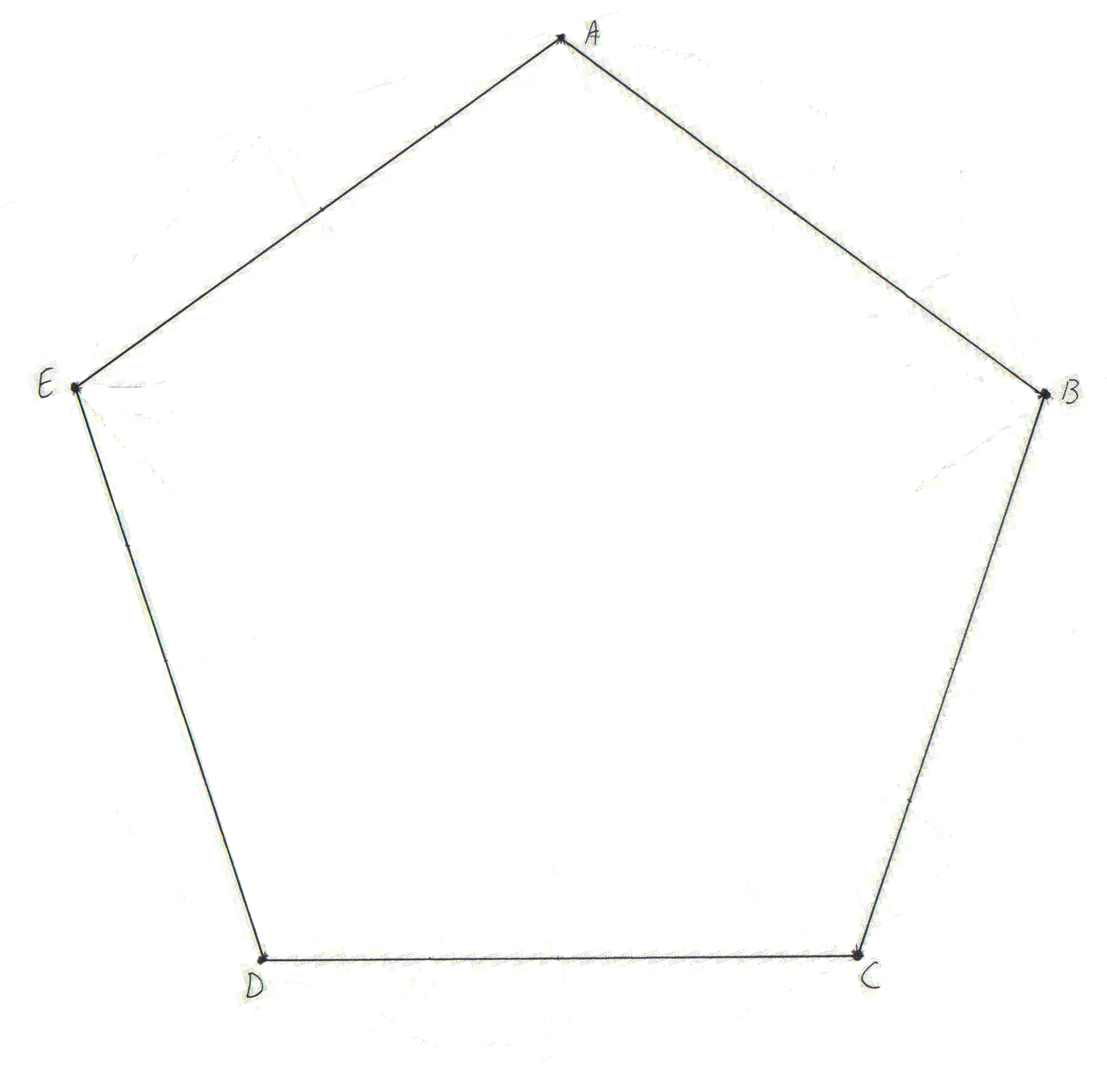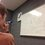# The Golden Ratio: Pentagonal DiagonalsHere is the previous post concerning the Golden Ratio. For a collection of all the posts concerning the Golden Ratio, click #GoldenRatio below.

We all know that the diagonal of a unit square (a square with sidelength 1) is $\sqrt{2}$. We could easily figure this out using the Pythagorean theorem. Then I ask you, what is the length of the diagonal of a regular unit pentagon? Try to solve this before moving on.

As you may have guessed, it is the golden ratio! But how did we figure it out? Well, take the pentagon pictured above. There are a lot of ways to solve this problem, but some require more algebra than others. Other techniques used could be the Law of Cosines or Ptolmey's Theorem. Here, we will just use good old geometry. You may want to draw a picture for yourself to follow what's happening.

Note that since we have a regular pentagon, $AB = BC = CD = DE = EA$. Also, because of its symmetry, all of its diagonals are equal, i.e. $AC = BD = CE = DA = EB$. Define point $F$ as the intersection of the lines $AC$ and $BD$ and draw $AC$ and $BD$. Now, let's do some angle chasing.

1. We know, or could easily calculate, that the interior angles of a pentagon are $\angle ABC = 108^{\circ}$
2. $\Delta ABC$ is isoceles because $AB = BC$
3. $\angle BAC = \angle BCA = 36^{\circ}$ because $\Delta ABC$ is isoceles
4. Using the same logic on $\Delta BCD$, $\angle DBC = \angle FBC = 36^{\circ}$
5. By the AA theorem, $\Delta ABC \sim \Delta BFC$
6. $\frac{CF}{BC} = \frac{BC}{AC}$ due to similarity. *
7. $\angle ABF = 108^{\circ}-36^{\circ} = 72^{\circ}$
8. $\angle BFA = 72^{\circ}$
9. $\Delta ABF$ is isoceles because $\angle ABF = \angle BFA$
10. $AB = AF$ *

Especially note steps 6 and 10. From there,

$\frac{AC}{AB} = \frac{AF + CF}{AB} = \frac{AF}{AB} + \frac{CF}{AB} = \frac{AF}{AB} + \frac{CF}{BC} = 1 + \frac{BC}{AC} = 1 + \frac{AB}{AC}$

This should start to look familiar. If not, let $x = \frac{AC}{AB}$. Then $x = 1 + \frac{1}{x}$. Then $x$ is the golden ratio! Thus, for a regular unit pentagon, the diagonals have length $\phi$.

Can you supply your own proof of this property of the pentagon? Don't be afraid to use algebra. What else is special about this pentagon?

Here is the next note.Note by Bob Krueger
7 years ago

This discussion board is a place to discuss our Daily Challenges and the math and science related to those challenges. Explanations are more than just a solution — they should explain the steps and thinking strategies that you used to obtain the solution. Comments should further the discussion of math and science.

When posting on Brilliant:

• Use the emojis to react to an explanation, whether you're congratulating a job well done , or just really confused .
• Ask specific questions about the challenge or the steps in somebody's explanation. Well-posed questions can add a lot to the discussion, but posting "I don't understand!" doesn't help anyone.
• Try to contribute something new to the discussion, whether it is an extension, generalization or other idea related to the challenge.

MarkdownAppears as
*italics* or _italics_ italics
**bold** or __bold__ bold
- bulleted- list
• bulleted
• list
1. numbered2. list
1. numbered
2. list
Note: you must add a full line of space before and after lists for them to show up correctly
paragraph 1paragraph 2

paragraph 1

paragraph 2

[example link](https://brilliant.org)example link
> This is a quote
This is a quote
    # I indented these lines
# 4 spaces, and now they show
# up as a code block.

print "hello world"
# I indented these lines
# 4 spaces, and now they show
# up as a code block.

print "hello world"
MathAppears as
Remember to wrap math in $$ ... $$ or $ ... $ to ensure proper formatting.
2 \times 3 $2 \times 3$
2^{34} $2^{34}$
a_{i-1} $a_{i-1}$
\frac{2}{3} $\frac{2}{3}$
\sqrt{2} $\sqrt{2}$
\sum_{i=1}^3 $\sum_{i=1}^3$
\sin \theta $\sin \theta$
\boxed{123} $\boxed{123}$